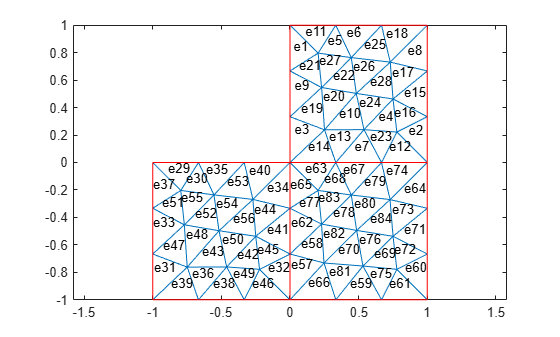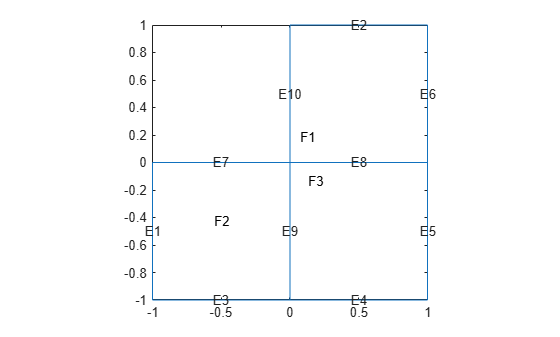# pdemesh

## Syntax

``pdemesh(model)``
``pdemesh(fegeometry)``
``pdemesh(mesh)``
``pdemesh(nodes,elements)``
``pdemesh(model,u)``
``pdemesh(___,Name,Value)``
``pdemesh(p,e,t)``
``pdemesh(p,e,t,u)``
``h = pdemesh(___)``

## Description

example

````pdemesh(model)` plots the mesh contained in a 2-D or 3-D `model` object.```
````pdemesh(fegeometry)` plots the mesh represented by the `Mesh` property of an `fegeometry` object.```

example

````pdemesh(mesh)` plots the mesh represented by an `FEMesh` object.```

example

````pdemesh(nodes,elements)` plots the mesh defined by `nodes` and `elements`.```

example

````pdemesh(model,u)` plots solution data `u` as a 3-D plot. This syntax is valid only for 2-D geometry.```

example

````pdemesh(___,Name,Value)` plots the mesh or solution data using any of the arguments in the previous syntaxes and one or more `Name,Value` pair arguments.```

example

````pdemesh(p,e,t)` plots the mesh specified by the mesh data `p`,`e`,`t`.```

example

````pdemesh(p,e,t,u)` plots PDE node or triangle data `u` using a mesh plot. The function plots the node data if `u` is a column vector , and triangle data if `u` is a row vector.If you want to have more control over your mesh plot, use `pdeplot` or `pdeplot3D` instead of `pdemesh`.```
````h = pdemesh(___)` returns handles to the graphics, using any of the arguments of the previous syntaxes.```

## Examples

collapse all

Create a mesh plot and display the node and element labels of the mesh.

Create a PDE model. Include the geometry of the built-in function `lshapeg`. Mesh the geometry.

```model = createpde; geometryFromEdges(model,@lshapeg); mesh = generateMesh(model);```

Plot the mesh.

`pdemesh(model)`Alternatively, you can plot a mesh by using `mesh` as an input argument.

`pdemesh(mesh)`Another approach is to use the nodes and elements of the mesh as input arguments for `pdemesh`.

`pdemesh(mesh.Nodes,mesh.Elements)`Display node labels.

`pdemesh(model,NodeLabels="on")`Use `xlim` and `ylim` to zoom in on particular nodes.

```xlim([-0.4,0.4]) ylim([-0.4,0.4])```Display element labels.

```pdemesh(model,ElementLabels="on") xlim([-0.4,0.4]) ylim([-0.4,0.4])```Apply boundary conditions, specify coefficients, and solve the PDE.

```applyBoundaryCondition(model,"dirichlet", ... Edge=1:model.Geometry.NumEdges, ... u=0); specifyCoefficients(model,m=0,... d=0,... c=1,... a=0,... f=1); generateMesh(model); results = solvepde(model)```
```results = StationaryResults with properties: NodalSolution: [1177x1 double] XGradients: [1177x1 double] YGradients: [1177x1 double] ZGradients: [] Mesh: [1x1 FEMesh] ```
`u = results.NodalSolution;`

Plot the solution at nodal locations by using `pdemesh`.

`pdemesh(model,u)`The `pdemesh` function ignores `NodeLabels` and `ElementLabels` when you plot solution data as a 3-D plot.

Create a PDE model, include the geometry and mesh it.

```model = createpde; importGeometry(model,"Plate10x10x1.stl"); generateMesh(model,Hmax=5);```

Plot the mesh setting the transparency to 0.5.

`pdemesh(model,FaceAlpha=0.5)`Find the elements associated with a geometric region.

Create a PDE model.

`model = createpde;`

Include the geometry of the built-in function `lshapeg`. Plot the geometry.

```geometryFromEdges(model,@lshapeg); pdegplot(model,FaceLabels="on",EdgeLabels="on")```Generate a mesh.

`mesh = generateMesh(model,Hmax=0.5);`

Find the elements associated with face 2.

`Ef2 = findElements(mesh,"region",Face=2);`

Highlight these elements in green on the mesh plot.

```figure pdemesh(mesh,ElementLabels="on") hold on pdemesh(mesh.Nodes,mesh.Elements(:,Ef2),EdgeColor="green")```Plot the mesh for the geometry of the L-shaped membrane.

```[p,e,t] = initmesh("lshapeg"); [p,e,t] = refinemesh("lshapeg",p,e,t); pdemesh(p,e,t)```Now solve Poisson's equation $-\Delta u=1$ over the geometry defined by the L-shaped membrane. Use Dirichlet boundary conditions $u=0$ on $\delta \Omega$, and plot the result.

```u = assempde("lshapeb",p,e,t,1,0,1); pdemesh(p,e,t,u)```## Input Arguments

collapse all

Model container, specified as an `femodel` object, `PDEModel` object, `ThermalModel` object, `StructuralModel` object, or `ElectromagneticModel` object.

PDE solution, specified as a vector or matrix.

Example: `results = solvepde(model); u = results.NodalSolution;` or ```u = assempde(model,c,a,f);```

Geometry object for finite element analysis, specified as an `fegeometry` object. The `pdemesh` function plots the mesh from the `Mesh` property of the `fegeometry` object.

Mesh description, specified as an `FEMesh` object. See FEMesh Properties.

Nodal coordinates, specified as a 2-by-NumNodes matrix for a 2-D mesh and 3-by-NumNodes matrix for a 3-D mesh. NumNodes is the number of nodes.

Element connectivity matrix in terms of node IDs, specified as an NodesPerElem-by-NumElements matrix. NodesPerElem is the number of nodes per element. Linear meshes contain only corner nodes, so there are three nodes per a 2-D element and four nodes per a 3-D element. Quadratic meshes contain corner nodes and nodes in the middle of each edge of an element. For quadratic meshes, there are six nodes per a 2-D element and 10 nodes per a 3-D element.Mesh points, specified as a 2-by-`Np` matrix of points, where `Np` is the number of points in the mesh. For a description of the (`p`,`e`,`t`) matrices, see Mesh Data as [p,e,t] Triples.

Typically, you use the `p`, `e`, and `t` data exported from the PDE Modeler app, or generated by `initmesh` or `refinemesh`.

Example: `[p,e,t] = initmesh(gd)`

Data Types: `double`

Mesh edges, specified as a `7`-by-`Ne` matrix of edges, where `Ne` is the number of edges in the mesh. For a description of the (`p`,`e`,`t`) matrices, see Mesh Data as [p,e,t] Triples.

Typically, you use the `p`, `e`, and `t` data exported from the PDE Modeler app, or generated by `initmesh` or `refinemesh`.

Example: `[p,e,t] = initmesh(gd)`

Data Types: `double`

Mesh triangles, specified as a `4`-by-`Nt` matrix of triangles, where `Nt` is the number of triangles in the mesh. For a description of the (`p`,`e`,`t`) matrices, see Mesh Data as [p,e,t] Triples.

Typically, you use the `p`, `e`, and `t` data exported from the PDE Modeler app, or generated by `initmesh` or `refinemesh`.

Example: `[p,e,t] = initmesh(gd)`

Data Types: `double`

### Name-Value Arguments

Specify optional pairs of arguments as `Name1=Value1,...,NameN=ValueN`, where `Name` is the argument name and `Value` is the corresponding value. Name-value arguments must appear after other arguments, but the order of the pairs does not matter.

Before R2021a, use commas to separate each name and value, and enclose `Name` in quotes.

Example: `pdemesh(model,NodeLabels="on")`

Node labels, specified as `"off"` or `"on"`.

`pdemesh` ignores `NodeLabels` when you plot solution data as a 3-D plot.

Example: `NodeLabels="on"`

Data Types: `char` | `string`

Element labels, specified as `"off"` or `"on"`.

`pdemesh` ignores `ElementLabels` when you plot solution data as a 3-D plot.

Example: `ElementLabels="on"`

Data Types: `char` | `string`

Surface transparency for 3-D geometry, specified as a real number from `0` through `1`. The default value `1` indicates no transparency. The value `0` indicates complete transparency.

Example: `FaceAlpha=0.5`

Data Types: `double`

Color of mesh edges, specified as a short or long color name or an RGB triplet. By default, for 2-D meshes the edges within one face are blue (RGB triplet `[0 0 1]`) and the edges between faces are red (RGB triplet `[1 0 0]`). For 3-D meshes, the default edge color is black (RGB triplet ```[0 0 0]```).

The short names and long names are character vectors that specify one of eight predefined colors. The RGB triplet is a three-element row vector whose elements specify the intensities of the red, green, and blue components of the color; the intensities must be in the range [0 1]. The following table lists the predefined colors and their RGB triplet equivalents.

RGB Triplet

Short Name

Long Name

`[1 1 0]`

`y`

`yellow`

`[1 0 1]`

`m`

`magenta`

`[0 1 1]`

`c`

`cyan`

`[1 0 0]`

`r`

`red`

`[0 1 0]`

`g`

`green`

`[0 0 1]`

`b`

`blue`

`[1 1 1]`

`w`

`white`

`[0 0 0]`

`k`

`black`

Example: `EdgeColor="green"`

Data Types: `double` | `char` | `string`

Color of mesh faces for 3-D meshes, specified as a short or long color name or an RGB triplet. The default face color is cyan (RGB triplet `[0 1 1]`). For details about available colors, see EdgeColor.

Example: `FaceColor="green"`

Data Types: `double` | `char` | `string`

## Output Arguments

collapse all

Handles to graphics objects, returned as a vector.

## Version History

Introduced before R2006a

expand all